Related Articles

# Filtering a row in PySpark DataFrame based on matching values from a list

• Last Updated : 28 Jul, 2021

In this article, we are going to filter the rows in the dataframe based on matching values in the list by using isin in Pyspark dataframe

isin(): This is used to find the elements contains in a given dataframe, it will take the elements and get the elements to match to the data

Syntax: isin([element1,element2,.,element n])

Create Dataframe for demonstration:

## Python3

 `# importing module``import` `pyspark`` ` `# importing sparksession``from` `pyspark.sql ``import` `SparkSession`` ` `# creating sparksession``# and giving an app name``spark ``=` `SparkSession.builder.appName(``'sparkdf'``).getOrCreate()`` ` `# list  of students  data  with null values``# we can define null values with none``data ``=` `[[``1``, ``"sravan"``, ``"vignan"``],``        ``[``2``, ``"ramya"``, ``"vvit"``],``        ``[``3``, ``"rohith"``, ``"klu"``],``        ``[``4``, ``"sridevi"``, ``"vignan"``],``        ``[``5``, ``"gnanesh"``, ``"iit"``]]`` ` `# specify column names``columns ``=` `[``'ID'``, ``'NAME'``, ``'college'``]`` ` `# creating a dataframe from the lists of data``dataframe ``=` `spark.createDataFrame(data, columns)`` ` `dataframe.show()`

Output: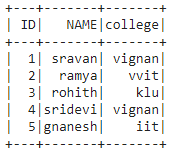## Method 1: Using filter() method

It is used to check the condition and give the results, Both are similar

Syntax: dataframe.filter(condition)

Where, condition is the dataframe condition.

Here we will use all the discussed methods.

Syntax: dataframe.filter((dataframe.column_name).isin([list_of_elements])).show()

where,

• column_name is the column
• elements are the values that are present in the column
• show() is used to show the resultant dataframe

Example 1: Get the particular ID’s with filter() clause.

## Python3

 `# get the ID : 1,2,3 from dataframe``dataframe.``filter``((dataframe.``ID``).isin([``1``,``2``,``3``])).show()`

Output: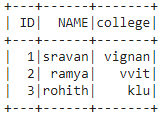Example 2: Get ID’s not present in 1 and 3

## Python3

 `# get the ID : not in 1 and 3 from dataframe``dataframe.``filter``(~(dataframe.``ID``).isin([``1``, ``3``])).show()`

Output: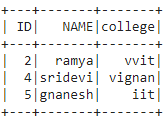Example 3: Get names from dataframe.

## Python3

 `# get name as sravan``dataframe.``filter``((``  ``dataframe.NAME).isin([``'sravan'``])).show()`

Output: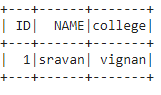## Method 2: Using where() method

where() is used to check the condition and give the results

Syntax: dataframe.where(condition)

where, condition is the dataframe condition

Overall Syntax with where clause:

dataframe.where((dataframe.column_name).isin([elements])).show()

where,

• column_name is the column
• elements are the values that are present in the column
• show() is used to show the resultant dataframe

Example: Get the particular colleges with where() clause

## Python3

 `# get college as vignan``dataframe.where((``  ``dataframe.college).isin([``'vignan'``])).show()`

Output: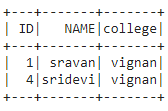Attention geek! Strengthen your foundations with the Python Programming Foundation Course and learn the basics.

To begin with, your interview preparations Enhance your Data Structures concepts with the Python DS Course. And to begin with your Machine Learning Journey, join the Machine Learning – Basic Level Course

My Personal Notes arrow_drop_up